Next: Electrostatics Up: Types of analysis Previous: Hydrodynamic lubrication   Contents

### Irrotational incompressible inviscid flow

If incompressible flow is irrotational a potential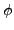exists for the velocity field such that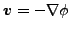. Furthermore, if the flow is inviscid one can prove that if a flow is irrotational at any instant in time, it remains irrotational for all subsequent time instants . The continuity equation now reads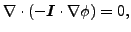(317)

and the balance of momentum for gravitational flow yields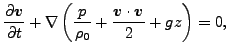(318)

where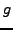is the earth acceleration,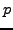is the pressure,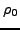is the density and z is the coordinate in earth direction. By comparison with the heat equation, the correspondence in Table  (12) arises.

 heat quantity irrotational flow quantity T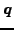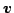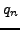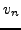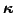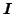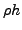0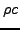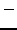Onceis determined, the velocityis obtained by differentiation and the pressurecan be calculated through the balance of momentum. Although irrotational incompressible inviscid flow sounds very special, the application field is rather large. Flow starting from rest is irrotational since the initial field is irrotational. Flow at speeds below 0.3 times the speed of sound can be considered to be incompressible. Finally, the flow outside the tiny boundary layer around an object is inviscid. A favorite examples is the flow around a wing profile. However, if the boundary layer separates and vortices arise the above theory cannot be used any more. For further applications see .Next: Electrostatics Up: Types of analysis Previous: Hydrodynamic lubrication   Contents
guido dhondt 2018-12-15